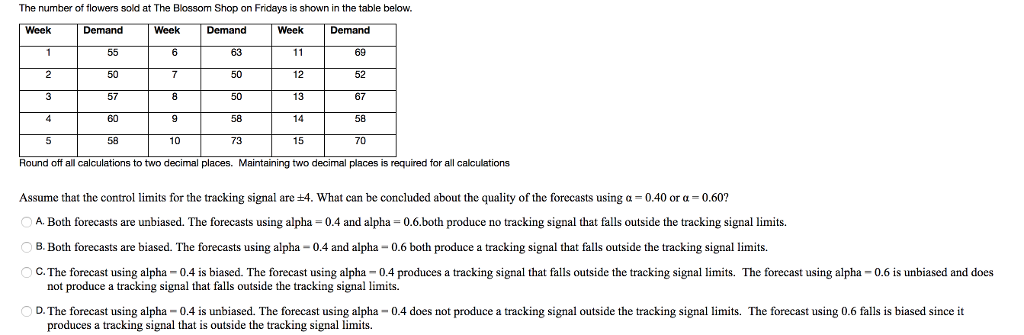# Question & Answer: The number of flowers sold at The Blossom Shop on Fridays is shown in the table…..

14.The number of flowers sold at The Blossom Shop on Fridays is shown in the table below. Round off all calculations to two decimal places. Maintaining two decimal places is required for all calculations Assume that the control limits for the tracking signal are plusminus 4. what can be concluded about the quality of the forecasts using alpha = 0.40 or alpha = 0.60? A. Both forecasts are unbiased. The forecasts using alpha = 0.4 and alpha = 0.6. both produce no tracking signal that falls outside the tracking signal limits. B. Both forecasts are biased. The forecasts using alpha = 0.4 and alpha = 0.6 both produce a tracking signal that falls outside the tracking signal limits. C. The forecast using alpha = 0.4 is biased. The forecast using alpha = 0.4 produces a tracking signal that falls outside the tracking signal limits. The forecast using alpha = 0.6 is unbiased and does not produce a tracking signal that falls outside the tracking signal limits. D. The forecast using al